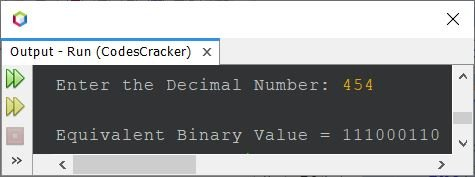# Java Program to Convert Decimal to Binary

This article covers multiple Java programs on the conversion of decimal to binary number. Here are the list of programs covered by this article:

• Decimal to binary conversion in Java using array
• Decimal to binary conversion in Java, completely using while loop
• Decimal to binary conversion in Java, completely using for loop
• Decimal to binary conversion in Java without using array

Note - If you're not aware about, how the decimal to binary conversion takes place, then refer to Decimal to Binary Conversion.

## Decimal to Binary in Java using Array

The question is, write a Java program to convert decimal number to binary. The decimal number must be received by user at run-time of the program. The program given below is the answer to this question:

```import java.util.Scanner;

public class CodesCracker
{
public static void main(String[] args)
{
int decimal, i=0;
int[] binary = new int;

Scanner scan = new Scanner(System.in);

System.out.print("Enter the Decimal Number: ");
decimal = scan.nextInt();

while(decimal != 0)
{
binary[i] = decimal%2;
i++;
decimal = decimal/2;
}

System.out.print("\nEquivalent Binary Value = ");
for(i=(i-1); i>=0; i--)
System.out.print(binary[i]);
}
}```

The snapshot given below shows the sample run of above program with user input 454 as decimal number to convert and prints its equivalent binary value:## Decimal to Binary in Java - Completely using while Loop

Since previous program uses both while and for loop. Therefore let's modify that program, to use only while loop, to do the same job, of converting decimal to binary number, with little other changes too.

```import java.util.Scanner;

public class CodesCracker
{
public static void main(String[] args)
{
Scanner scan = new Scanner(System.in);

System.out.print("Enter the Decimal Number: ");
int decimal = scan.nextInt();

int i=0;
int[] binary = new int;
while(decimal != 0)
{
binary[i] = decimal%2;
i++;
decimal /= 2;
}

System.out.print("\nEquivalent Binary Value = ");
i = (i-1);
while(i>=0)
{
System.out.print(binary[i]);
i--;
}
}
}```

You'll get the same output as of previous program.

## Decimal to Binary in Java - Completely using for Loop

This program is similar to previous program. The only difference is, the loop. That is, this program uses for loop, instead of while, to do the same job as of previous program.

```import java.util.Scanner;

public class CodesCracker
{
public static void main(String[] args)
{
Scanner scan = new Scanner(System.in);

System.out.print("Enter the Decimal Number: ");
int decimal = scan.nextInt();

int i;
int[] binary = new int;
for(i=0; decimal!=0; decimal/=2)
binary[i++] = decimal%2;

System.out.print("\nEquivalent Binary Value = ");
for(i=(i-1); i>=0; i--)
System.out.print(binary[i]);
}
}```

In above program, the statement:

`binary[i++] = decimal%2;`

is same as:

```binary[i] = decimal%2;
i++;```

That is, ++ after the variable i, uses the current value of i, then increments by 1.

## Decimal to Binary in Java without using Array

This is the last program of this article, created to convert decimal to binary number, without using array.

```import java.util.Scanner;

public class CodesCracker
{
public static void main(String[] args)
{
int decimal, binary=0, m=1, rem;
Scanner scan = new Scanner(System.in);

System.out.print("Enter the Decimal Number: ");
decimal = scan.nextInt();

while(decimal>0)
{
rem = decimal%2;
binary = binary + (rem*m);
m *= 10;
decimal /= 2;
}

System.out.print("\nEquivalent Binary Value = " +binary);
}
}```

#### Same Program in Other Languages

Java Online Test

« Previous Program Next Program »